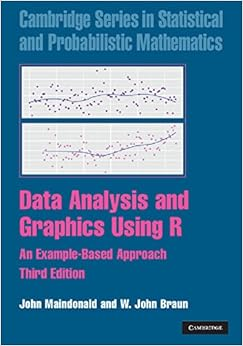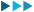Data Analysis and Graphics Using R: An Example-Based Approach (Cambridge Series in Statistical and Probabilistic Mathematics) 9780521762939 Biostatist

MRP: 7244.67
450037%

Enter your email id to set price alert:

Thank you for subscribing. You are just one more step away from getting price alerts. Please go to your email inbox & verify your email id.
View more deals from Othersx

Price Graph

1. Current price of Data Analysis and Graphics Using R: An Example-Based Approach (Cambridge Series in Statistical and Probabilistic Mathematics) 9780521762939 Biostatist is Rs. 4500
2. Price of Data Analysis and Graphics Using R: An Example-Based Approach (Cambridge Series in Statistical and Probabilistic Mathematics) 9780521762939 Biostatist has dropped to its minimum 126 days ago !
3. The lowest price for Data Analysis and Graphics Using R: An Example-Based Approach (Cambridge Series in Statistical and Probabilistic Mathematics) 9780521762939 Biostatist was noted on 2019-06-10 16:27:58
4. Data Analysis and Graphics Using R: An Example-Based Approach (Cambridge Series in Statistical and Probabilistic Mathematics) 9780521762939 Biostatist is not at it's all time low. You can wait for the price to drop again by setting an alert above. You will be notified as soon as the price drops !
5. Price of Data Analysis and Graphics Using R: An Example-Based Approach (Cambridge Series in Statistical and Probabilistic Mathematics) 9780521762939 Biostatist has varied from 1727 to 4500 in last 844 days.
6. Percentage Drop shown in the deal is w.r.t MRP of the product. It doesn't depict how good a particular deal is !